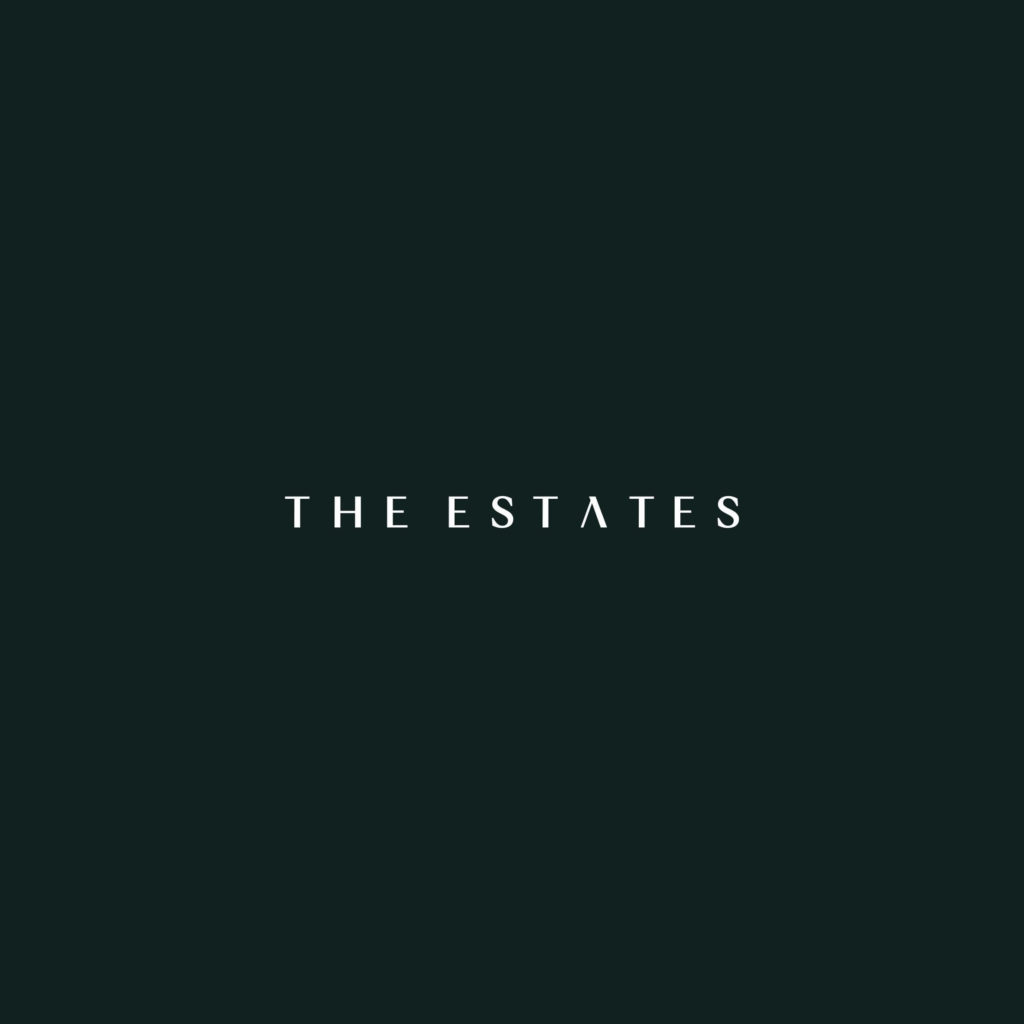# The Estates New Zayed

## The Estates New Zayed

The Estates New Zayed by sodic As one of our distinguished customers it is with pleasure that I invite you to an exclusive preview of our latest signature community; The Estates – located in New Zayed 5 mins away from SODIC west with unique contemporary country architecture and extended green views.

I N T R O D U C I N G
S O D I C
‘SIGNATURES’

W i t h h o m e s t a i l o r e d t o r e f l e c t y o u r s t y l e a n d p e r s o n a l i t y , ‘ S O D I C
S i g n a t u r e s’ t a k e s p r e m i u m l i v i n g t o a n e w l e v e l .

With integrated communities focused on what’s most important to you, you can rest easy knowing that you’re getting the most premium services available, whether it’s the spa, retail, or education options.

our residents only experience the best of the best. ‘SODIC Signatures’ introduces an unparalleled level of luxury and exclusive community management alongside the amenities, style, quality, and creature comforts that cement ‘Signatures’ as SODIC’s flagship developments.

T H E E S T A T E S :

L U X U R Y L I V I N G ,
REINVENTED

T h e E s t a t e s o f f e r s
y o u s o m e t h i n g
c o m p l e t e l y n e w , y e t
w i t h t r a d i t i o n a l
values at heart.

M O R E
T H A N

J U S T A
HOME:
A HAVEN…

S u r r o u n d e d b y t r e e s a n d g r e e n e r y , T h e
Estates is an oasis of calm and serenity.

P E R F E C T L Y
POSITIONED

The Estates i s l o c a t e d directly on the CairoA l e x a n d r i a D e s e r t R o a d , m o m e n t s a w a y f r o m t h e v i b r a n t , t h r i v i n g S O D I C We s t a n d m i n u t e f r o m S p h i n x International Airport.

2 minutes to SODIC West
3 minutes to Sphinx Airport
7 minutes to Mehwar
12 Minutes to Arkan

15 Minutes to Grand Museum
25 Minutes to Mohandiseen
50 Minutes to Heliopolis

H E A L T H Y ,
S A F E A N D
GREEN

A t T h e E s t a t e s w e f o c u s o n h e a l t h y o u t d o o r
a c t i v i t i e s , g e t t i n g y o u b a c k t o n a t u r e a n d t h e
enjoyment of the simple pleasures in life.

E X T R A O R D I N A R Y
H O M E S F O R
E X T R A O R D I N A R Y
PEOPLE

A h o m e a t The Estates
g u a r a n t e e s u n p a r a l l e l e d
s t y l e a n d r e f i n e m e n t .

R O O M
T O
RELAX

A l l h o m e s a r e w e l l s p a c e d f r o m o n e a n o t h e r a n d b l e n d
s e a m l e s s l y a n d t a s t e f u l l y i n t o t h e s u r r o u n d i n g o u t d o o r s p a c e s .